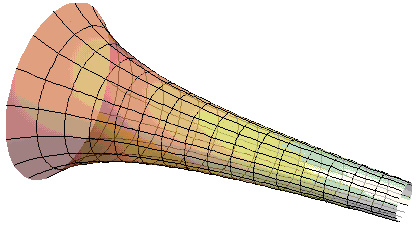# Volume and Area of Torricelli's Trumpet

In an article on Paradoxes of Infinity I mentioned a $3D$ figure known as Torricelli's Trumpet, also called Gabriel's Horn, whose surface area is infinite but whose volume is finite. Below I shall establish these facts.

Torricelli's Trumpet is the surface of revolution obtained by rotating the graph of the function $\displaystyle f(x)=\frac{1}{x}$ on the interval $[1,\infty)$ around the $x-\mbox{axis}.$The volume of Torricelli's Trumpet is given by the integral $\displaystyle V=\pi\int_{1}^{\infty}f^{2}(x)dx=\pi\int_{1}^{\infty}\frac{dx}{x^2};$ its area by the integral $\displaystyle S=2\pi\int_{1}^{\infty}f(x)\sqrt{1+[f'(x)]^{2}}dx=2\pi\int_{1}^{\infty}\frac{1}{x}\sqrt{1+\frac{1}{x^4}}dx.$ Both integrals are improper in that they are taken over infinite intervals. Improper integrals of this sort are, by definition, the limits of integrals over finite intervals: $\displaystyle\int_{1}^{\infty}=\lim_{a\rightarrow\infty}\int_{1}^{a}.$ If the limit does not exist (or is infinite) the improper integral is said to diverge, otherwise it's convergent.

The volume integral is the easier of the two: $\displaystyle V=\lim_{a\rightarrow\infty}\pi\int_{1}^{a}\frac{dx}{x^2}.$ Computing it gives

$\displaystyle V=\lim_{a\rightarrow\infty}\pi$(-\frac{1}{x})\bigg|_{1}^{a},$(-\frac{1}{x})\bigg|_{1}^{a}$,$(-\frac{1}{x^2})\bigg|_{1}^{a}$,$(-\frac{1}{x^3})\bigg|_{1}^{a}$$\displaystyle =\lim_{a\rightarrow\infty}\pi (1-\frac{1}{a}),(1-\frac{1}{a}),(\frac{1}{a}-1)$$=1.$

Concerning the area integral, to prove the claim that it is infinite, we do not actually need to calculate the integral but only to estimate its growth. This is not difficult:

$\displaystyle S=2\pi\lim_{x\rightarrow\infty}\int_{1}^{a}\frac{1}{x}\sqrt{1+\frac{1}{x^4}}dx\ge 2\pi\lim_{x\rightarrow\infty}$\displaystyle\int_{1}^{a}\frac{1}{x}dx,$\displaystyle\int_{1}^{a}\frac{1}{x^4}dx$,$\displaystyle\int_{1}^{a}\frac{1}{x}dx$

Thus, $\displaystyle S\ge 2\pi\lim_{x\rightarrow\infty}\ln x\bigg|_{1}^{a}=2\pi\lim_{x\rightarrow\infty}\ln a=\infty.$Copyright © 1996-2018 Alexander Bogomolny

68259714